## You measure the power delivered by a battery to be 1.15 W when it is connected in series with two equal resistors. How much power will the s

Question

You measure the power delivered by a battery to be 1.15 W when it is connected in series with two equal resistors. How much power will the same battery deliver if the resistors are now connected in parallel across it

in progress 0
5 months 2021-08-26T04:51:10+00:00 1 Answers 3 views 0

## Answers ( )

The power is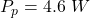Explanation:

From the question we are told that

The power delivered is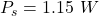Let it resistance be denoted as R

The resistors are connected in series so the equivalent resistance is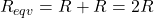Considering when it is connected in series

Generally power is mathematically represented as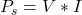Here I is the current which is mathematically represented as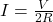The power becomes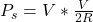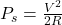substituting value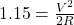Considering when resistance is connected in parallel

The equivalent resistance becomes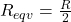So The current  becomes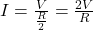And the power becomes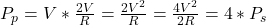substituting values